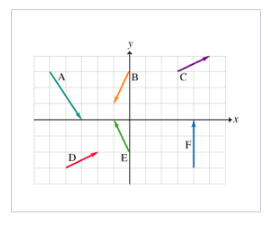# Problem: Six vectors ( A to F) have the magnitudes and directions indicated in the figure. Which two vectors, when subtracted (when one vector is subtracted from the other), will have the largest magnitude?1. A &amp; F2. A &amp; E3. D &amp; B4. C &amp; D5. E &amp; F

###### FREE Expert Solution

Vector magnitude:

$\overline{)\mathbf{|}\stackrel{\mathbf{⇀}}{\mathbit{a}}\mathbf{|}{\mathbf{=}}\sqrt{{{\mathbit{a}}_{\mathbit{x}}}^{\mathbf{2}}\mathbf{+}{{\mathbit{a}}_{\mathbit{y}}}^{\mathbf{2}}}}$

A = 2i - 3j

B = -1i - 2j

C = 2i + 1j

D = 2i + 1j

E = -1i + 2j

F = 0 i + 3j

Subtraction of vectors:

81% (446 ratings)###### Problem Details

Six vectors ( A to F) have the magnitudes and directions indicated in the figure.Which two vectors, when subtracted (when one vector is subtracted from the other), will have the largest magnitude?

1. A & F

2. A & E

3. D & B

4. C & D

5. E & F